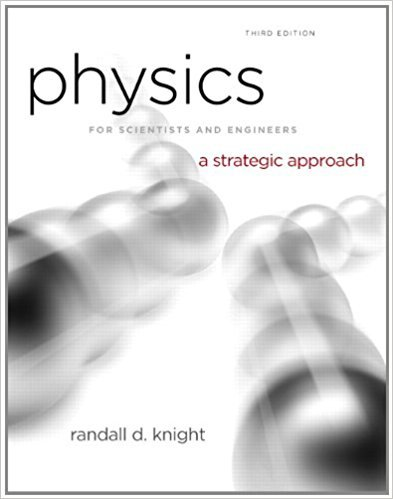×
Get Full Access to Physics For Scientists And Engineers: A Strategic Approach With Modern Physics - 3 Edition - Chapter 11 - Problem 60p
Get Full Access to Physics For Scientists And Engineers: A Strategic Approach With Modern Physics - 3 Edition - Chapter 11 - Problem 60p

×

# A clever engineer designs a “sprong” that obeys the forceISBN: 9780321740908 69

## Solution for problem 60P Chapter 11

Physics for Scientists and Engineers: A Strategic Approach with Modern Physics | 3rd Edition

• Textbook Solutions
• 2901 Step-by-step solutions solved by professors and subject experts
• Get 24/7 help from StudySoup virtual teaching assistantsPhysics for Scientists and Engineers: A Strategic Approach with Modern Physics | 3rd Edition

4 5 1 291 Reviews
28
3
Problem 60P

Problem 60P

A clever engineer designs a “sprong” that obeys the force law Fx =−q(x − xe)3, where xe is the equilibrium position of the end of the sprong and q is the sprong constant. For simplicity, we’ll let xe = 0 m. Then Fx = − qx3.

a. What are the units of q ?

b. Find an expression for the potential energy of a stretched or compressed sprong.

c. A sprong-loaded toy gun shoots a 20 g plastic ball. What is the launch speed if the sprong constant is 40,000, with the units you found in part a, and the sprong is compressed 10 cm? Assume the barrel is frictionless.

Step-by-Step Solution:

Solution

Step 1 of 3

1. We need to find out the unit of.

The unit ofis.

Step 2 of 3

Step 3 of 3

##### ISBN: 9780321740908

Since the solution to 60P from 11 chapter was answered, more than 3357 students have viewed the full step-by-step answer. Physics for Scientists and Engineers: A Strategic Approach with Modern Physics was written by and is associated to the ISBN: 9780321740908. The answer to “A clever engineer designs a “sprong” that obeys the force law Fx =?q(x ? xe)3, where xe is the equilibrium position of the end of the sprong and q is the sprong constant. For simplicity, we’ll let xe = 0 m. Then Fx = ? qx3.a. What are the units of q ?________________b. Find an expression for the potential energy of a stretched or compressed sprong.________________c. A sprong-loaded toy gun shoots a 20 g plastic ball. What is the launch speed if the sprong constant is 40,000, with the units you found in part a, and the sprong is compressed 10 cm? Assume the barrel is frictionless.” is broken down into a number of easy to follow steps, and 107 words. This textbook survival guide was created for the textbook: Physics for Scientists and Engineers: A Strategic Approach with Modern Physics, edition: 3. This full solution covers the following key subjects: sprong, compressed, Units, constant, launch. This expansive textbook survival guide covers 17 chapters, and 1439 solutions. The full step-by-step solution to problem: 60P from chapter: 11 was answered by , our top Physics solution expert on 08/30/17, 04:34AM.

Unlock Textbook Solution